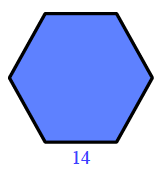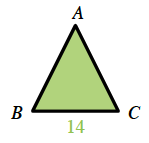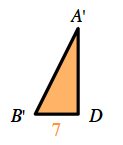### Home > CCG > Chapter 9 > Lesson 9.1.3 > Problem9-34

9-34.

A regular hexagonal prism has a volume of $2546.13\text{ cm}^3$and the base has an edge length of $14$ cm. Find the height and surface area of the prism.

Start by finding the area of the base of the prismDivide the hexagon into six triangles.

Find $∠A$ by dividing the central angle of the hexagon by $6$.

$\frac{360°}{6\text{ triangles}}=60°$

$m∠A = 60°$Divide the triangles in half to make two right triangles.

Solve for unknown angles. Is this a special triangle?

$\angle A'=\frac{60°}{2}$

$m∠A' = 30°$When you find the area of the $30\text{-}60\text{-}90$ triangle, multiply it by $12$ in order to find the area of the hexagon.

$\angle B'=180°-30°-90°$

$m∠B' = 60°$

$\text{Volume}=(\text{area of the base})(\text{height})$
$2546.12\text{ cm}^³ = (509.23\text{ cm}^²)(\text{height})$

Once you have the height of the prism, you have all the information necessary to find the surface area.